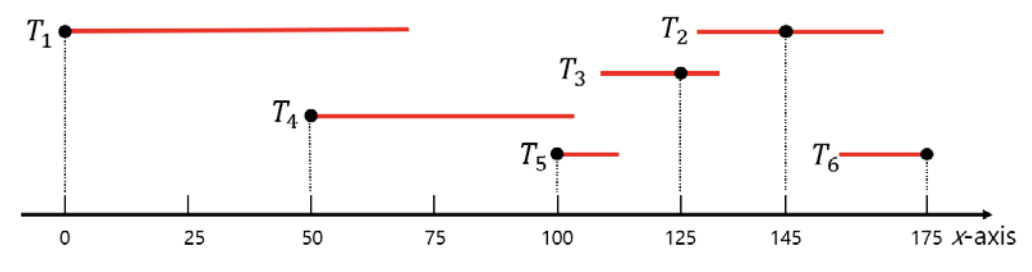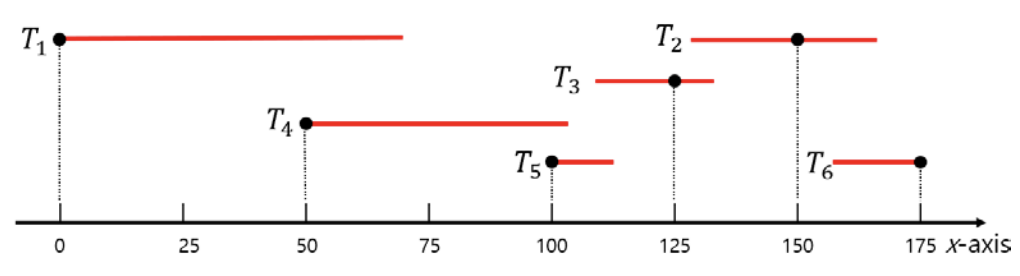시간 제한메모리 제한제출정답맞힌 사람정답 비율
1 초 512 MB89732021330.692%

## 문제

There are n threads on the x-axis. The length of the i-th thread, say Ti, is denoted by li and the location of its starting point by xi, both being integers. We want to make a knot in each thread. The location of the knot must also be an integer. The knot can be made at any point in the thread and it is assumed that the length of the thread is not reduced by the knot. You can assume that no thread is totally contained by another, which means that there are no two thread Ti and Tj (ij) such that xjxi and xi+lixj+lj.

We want to determine the location of the knot for each thread in order to make the distance between the closest two neighboring knots as big as possible.

For example, the figures below show the locations of the knots for six threads. The location of a knot is denoted by a point. All the threads actually lie on the x-axis, however, they are drawn separately to distinguish each other. In Figure I.1, the distance between the closest two knots is 20. However, if we make the knot for T2 at different location as shown in Figure I.2, the distance between the closest two knots becomes 25, which is what this problem requests.Figure I.1: An example of knots for 6 threads.Figure I.2: Another example with different location of knot for T2.

Given information about the n threads, write a program that calculates the maximum distance between two closest knots.

## 입력

Your program is to read from standard input. The input starts with a line containing one integer, n (2 ≤ n ≤ 100,000), where n is the number of threads. In the following n lines, the i-th line contains two integers xi (0 ≤ xi ≤ 109) and li (1 ≤ li ≤ 109), where xi and li denote the location of the starting point and the length of the i-th thread, respectively, You can assume that no thread is totally contained by another, which means that there are no two threads Ti and Tj (ij) such that xjxi and xi+lixj+lj.

## 출력

Your program is to write to standard output. Print exactly one line. The line should contain an integer which is the maximum distance between two closest knots.

## 예제 입력 1

6
0 67
127 36
110 23
50 51
100 12
158 17


## 예제 출력 1

25


## 예제 입력 2

6
0 40
10 55
45 28
90 40
83 30
120 30


## 예제 출력 2

30


## 예제 입력 3

3
0 20
40 10
100 20


## 예제 출력 3

50